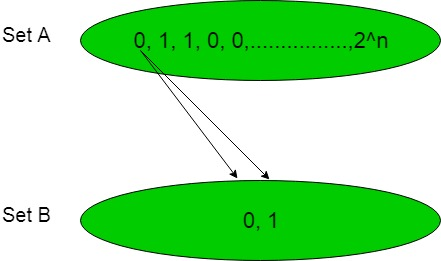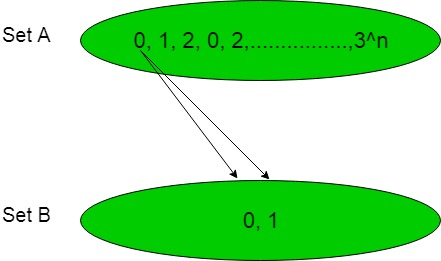# Digital Logic | Number of Boolean functions

In the below article, we are going to find the number of Boolean Functions possible from the given sets of binary number.

Statement-1:
Suppose two sets are set ‘A’ = {1, 2, 3, 4, …….., n} where each number will be either ‘0’ or ‘1’ and hence the total number of boolean variable possible will beand set ‘B’ = {0, 1}. Now the number of possible boolean function when counting is done from set ‘A’ to ‘B’ will be.

Explanation:
As we know that boolean variable is either ‘0’ or ‘1’ and in the set ‘A’ there are ‘n’ numbers and each number will be either ‘0’ or ‘1’ and hence the total number of possible boolean variable are. Now set ‘A’ containboolean variable and set ‘B’ contain 2 boolean variable.

This can be understood with help of below diagram:Each element of set ‘A’ makes a function with each element of set ‘B’ hence one element of set ‘A’ makes two function with set ‘B’ and hence total number of possible boolean functions arewhereis the number of element in set ‘A’.

Statement-2:
Suppose two sets of ternary variable are set ‘A’ = {1, 2, 3, 4, …….., n} where each number will be either ‘0’ or ‘1’ or ‘2’ and hence the total number of ternary variable possible will beand set ‘B’ = {0, 1}. Now the number of possible boolean function when counting is done from set ‘A’ to ‘B’ will be.

Explanation:
As we know that ternary variables are ‘0’ or ‘1’ or ‘2’ and in the set ‘A’ there are ‘n’ numbers and each number will be either ‘0’ or ‘1’ or ‘2’ and hence the total number of possible ternary variables are. Now set ‘A’ containternary numbers and set ‘B’ contain 2 boolean variable.

This can be understood with help of below diagram:Each element of set ‘A’ makes a function with each element of set ‘B’ hence one element of set ‘A’ makes two functions with set ‘B’ and hence the total number of possible boolean functions arewhereis the number of elements in set ‘A’.

Note:

Similarly for a set ‘A’ of ‘n’ numbers of k-ary variable and set ‘B’ of p-ary variable then the total number of p-ary possible function will be.My Personal Notes arrow_drop_upCheck out this Author's contributed articles.

If you like GeeksforGeeks and would like to contribute, you can also write an article using contribute.geeksforgeeks.org or mail your article to contribute@geeksforgeeks.org. See your article appearing on the GeeksforGeeks main page and help other Geeks.

Please Improve this article if you find anything incorrect by clicking on the "Improve Article" button below.

1

Please write to us at contribute@geeksforgeeks.org to report any issue with the above content.